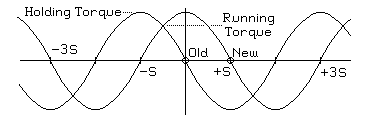Electronic Circuits and Tutorials
Tutorials
Dictionaries
Projects
Discover
STEPPING MOTOR PHYSICS TUTORIALS

# Dynamics

Each time you step the motor, you electronically move the equilibrium position S radians. This moves the entire curve illustrated in Figure 2.1 a distance of S radians, as shown in Figure 2.6:Figure 2.6

The first thing to note about the process of taking one step is that the maximum available torque is at a minimum when the rotor is halfway from one step to the next. This minimum determines the running torque, the maximum torque the motor can drive as it steps slowly forward. For common two-winding permanent magnet motors with ideal sinusoidal torque versus position curves and holding torque h, this will be h/(20.5). If the motor is stepped by powering two windings at a time, the running torque of an ideal two-winding permanent magnet motor will be the same as the single-winding holding torque.

It should be noted that at higher stepping speeds, the running torque is sometimes defined as the pull-out torque. That is, it is the maximum frictional torque the motor can overcome on a rotating load before the load is pulled out of step by the friction. Some motor data sheets define a second torque figure, the pull-in torque. This is the maximum frictional torque that the motor can overcome to accelerate a stopped load to synchronous speed. The pull-in torques documented on stepping motor data sheets are of questionable value because the pull-in torque depends on the moment of inertia of the load used when they were measured, and few motor data sheets document this!

In practice, there is always some friction, so after the equilibrium position moves one step, the rotor is likely to oscillate briefly about the new equilibrium position. The resulting trajectory may resemble the one shown in Figure 2.7:Figure 2.7

Here, the trajectory of the equilibrium position is shown as a dotted line, while the solid curve shows the trajectory of the motor rotor.